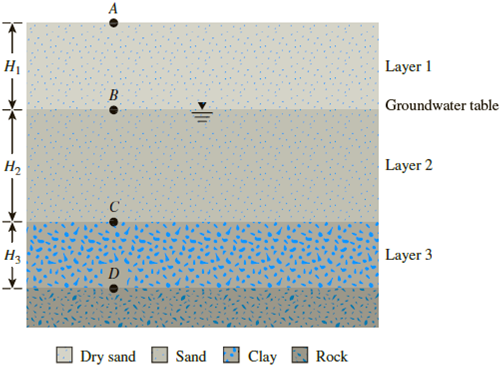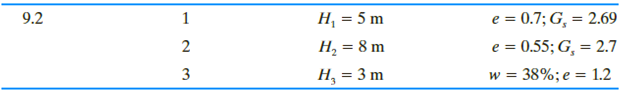Chapter 9, Problem 9.2PPrinciples of Geotechnical Enginee...

9th Edition
Braja M. Das + 1 other
ISBN: 9781305970939

Solutions

Chapter
SectionPrinciples of Geotechnical Enginee...

9th Edition
Braja M. Das + 1 other
ISBN: 9781305970939
Textbook Problem

9.1 Through 9.3 A soil profile consisting of three layers is shown in Figure 9.25. Calculate the values of σ, u, and σ′ at points A, B, C, and D for the following cases. In each case, plot the variations of σ, u, and σ′ with depth. Characteristics of layers 1, 2, and 3 for each case are given below:Figure 9.25To determine

Find the total stress σ , pore water pressure u , and effective stress σ at points A, B, C, and D and plot the variations of total stress, pore water pressure, and effective stress.

Explanation

Given information:

The thickness H1 of soil layer 1 is 5 m.

The thickness H2 of soil layer 2 is 8 m.

The thickness H3 of soil layer 3 is 3 m.

The void ratio (e) of soil in the first layer is 0.7.

The specific gravity Gs of the soil in the first layer is 2.69.

The void ratio (e) of soil in the second layer is 0.55.

The specific gravity Gs of the soil in the second layer is 2.7.

The water content (w) of the soil in the third layer is 38 %.

The void ratio (e) of the soil in the third layer is 1.2.

Calculation:

Determine the dry unit weight γd of the soil in the first layer using the relation.

γd=Gsγw1+e

Here, γw is the unit weight of the water.

Take the unit weight of the water as 9.81kN/m3.

Substitute 2.69 for Gs, 9.81kN/m3 for γw, and 0.7 for e.

γd=2.69×9.811+0.7=15.52kN/m3

Determine the saturated unit weight γsat of the soil in the second layer using the relation.

γsat=γw(Gs+e)1+e

Substitute 9.81kN/m3 for γw, 2.7 for Gs, and 0.55 for e.

γsat=9.81(2.7+0.55)1+0.55=20.57kN/m3

Determine the saturated unit weight γsat of the soil in the third layer using the relation.

γsat=γw(Gs+e)1+eγsat=γw(ew+e)1+e

Substitute 9.81kN/m3 for γw, 1.2 for e, and 38 % for w.

γsat=9.81(1.2(38100)+1.2)1+1.2=19.43kN/m3

Calculate the total stress at point A (0 m).

σ=0

Thus, the total stress at point A is zero_.

Calculate the pore water pressure at point A (0 m).

u=0

Thus, the pore water pressure at point A is zero_.

Calculate the effective stress at point A (0 m) using the relation.

σ=σu

Substitute 0 for σ and 0 for u.

σ=00=0

Thus, the effective stress at point A is zero_.

Calculate the total stress at point B (5 m) using the relation.

σ=γd×H1

Substitute 15.52kN/m3 for γd and 5 m for H1.

σ=15.52×5.0=77.6kN/m2

Thus, the total stress at point B is 77.6kN/m2_.

Calculate the pore water pressure at point B (5 m) using the relation.

u=0

Thus, the pore water pressure at point B is zero_.

Calculate the effective stress at point B (5 m) using the relation.

σ=σu

Substitute 77.6kN/m2 for σ and 0 for u.

σ=77.60=77.6kN/m2

Thus, the effective stress at point B is 77.6kN/m2_

Still sussing out bartleby?

Check out a sample textbook solution.

See a sample solution

The Solution to Your Study Problems

Bartleby provides explanations to thousands of textbook problems written by our experts, many with advanced degrees!

Get Started

What do we mean by a uniform series payment?

Engineering Fundamentals: An Introduction to Engineering (MindTap Course List)

What is a DBMS, and what are its functions?

Database Systems: Design, Implementation, & Management

Explain how to use a micrometer to measure cam lobe wear.

Automotive Technology: A Systems Approach (MindTap Course List)

Explain why database design is important.

Database Systems: Design, Implementation, & Management

What is game theory? Identify two applications of game theory.

Principles of Information Systems (MindTap Course List)

List at least three hardware devices listed in the System Report or in the Device Manager.

Enhanced Discovering Computers 2017 (Shelly Cashman Series) (MindTap Course List)

How does a welding transformer work?

Welding: Principles and Applications (MindTap Course List)